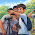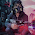## Friday, November 18, 2011

### Boolean Algebra & Logic Gates for A/L ICTBoolean algebra or Boolean logic is a calculation of truth values, developed by George Boole in the 1840s. In ICT it resembles logical multiplication xy, addition x + y, and negation −x to build combined circuits.

Boolean logic or Boolean algebra is a logical calculus which has only two values, 'true' or 'false'.
Boolean Logic is defined as a logical system of operators - 'AND', 'OR' and 'NOT'. It is a way of comparing individual bits and used in computer construction, switching circuits, etc.

' denotes negative
Boolean Algebra Quiz

1. Give the relationship that represents the dual of the Boolean property A + 1 = 1?
(Note: * = AND, + = OR and ' = NOT)
1. A * 1 = 1
2. A * 0 = 0
3. A + 0 = 0
4. A * A = A
5. A * 1 = 1

2. Give the best definition of a literal?
1. A Boolean variable
2. The complement of a Boolean variable
3. 1 or 2
4. A Boolean variable interpreted literally
5. The actual understanding of a Boolean variable

3. Simplify the Boolean expression (A+B+C)(D+E)' + (A+B+C)(D+E) and choose the best answer.
1. A + B + C
2. D + E
3. A'B'C'
4. D'E'
5. None of the above

4.Which of the following relationships represents the dual of the Boolean property x + x'y = x + y?
1. x'(x + y') = x'y'
2. x(x'y) = xy
3. x*x' + y = xy
4. x'(xy') = x'y'
5. x(x' + y) = xy

5.Given the function F(X,Y,Z) = XZ + Z(X'+ XY), the equivalent most simplified Boolean representation for F is:
1. Z + YZ
2. Z + XYZ
3. XZ
4. X + YZ
5. None of the above

6. Simplification of the Boolean expression (A + B)'(C + D + E)' + (A + B)' yields which of the following results?
1. A + B
2. A'B'
3. C + D + E
4. C'D'E'
5. A'B'C'D'E'

7. Given that F = A'B'+ C'+ D'+ E', which of the following represent the only correct expression for F'?
1. F'= A+B+C+D+E
2. F'= ABCDE
3. F'= AB(C+D+E)
4. F'= AB+C'+D'+E'
5. F'= (A+B)CDE

8. An equivalent representation for the Boolean expression A' + 1 is
1. A
2. A'
3. 1
4. 0

9. Simplification of the Boolean expression AB + ABC + ABCD + ABCDE + ABCDEF yields which of the following results?
1. ABCDEF
2. AB
3. AB + CD + EF
4. A + B + C + D + E + F
5. A + B(C+D(E+F))

1. A * 0 = 0. The dual of any Boolean property may be obtained by interchanging all occurrences of the symbols (*,+,0,1) with the symbols (+,*,1,0). Beware of implicit AND operations (like xy); these ANDs are also converted to +. Therefore, the dual of x + 1 = 1 is: x*0 = 0.

2. 1 or 2
3. A + B + C
4. x(x' + y) = xy
5. Z + XYZ

6. A'B'
7. (A+B)CDE (DeMorgan's theorem twice)
8. 1
9. AB

1.I am here to discuss a simple definition of Boolean logic as-Boolean logic is a system of symbolic logic which is used in computers.Study of mathematical operations performed on binary variables that can have only two values: true or false. It provides a set of rules called Boolean logic that are indispensable in digital computer-circuit and switching-circuit design.
Boolean Operators

2.3.4.5.6.